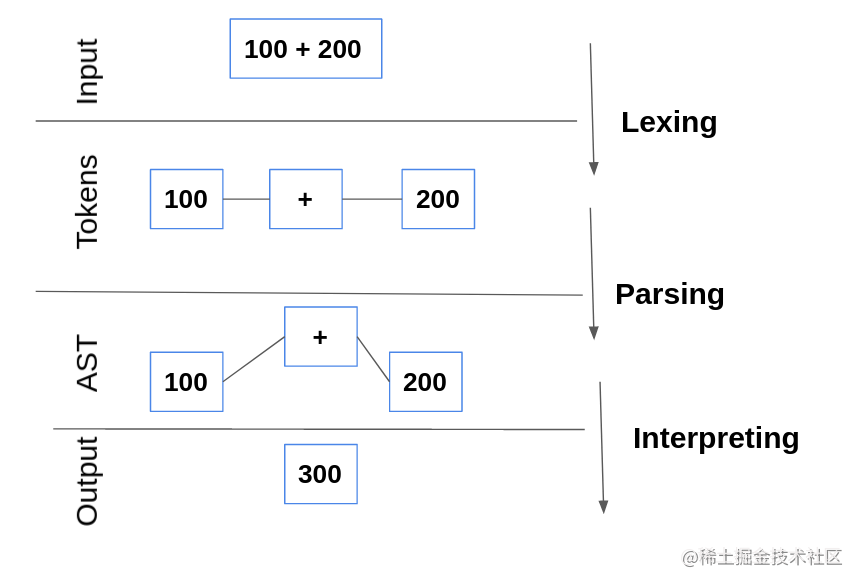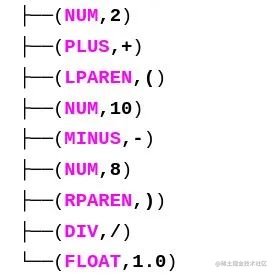# 使用 Python 开发一个 Python 解释器

PLY 可以通过以下方式下载：

`\$ pip install ply`## 标记（Token）

`标记`是为解释器提供有意义信息的最小字符单位。标记包含一对名称和属性值。

```tokens = (     # 数据类型     "NUM",     "FLOAT",     # 算术运算     "PLUS",     "MINUS",     "MUL",     "DIV",     # 括号     "LPAREN",     "RPAREN", )```

```

# 标记的正则表达

t_PLUS   = r"+" t_MINUS  = r"-" t_MUL    = r"*" t_DIV    = r"/" t_LPAREN = r"(" t_RPAREN = r")" t_POW    = r"^"

t_ignore = " \t"

# 为每个规则添加动作

def t_FLOAT(t):     r"""\d+.\d+"""     t.value = float(t.value)     return t

def t_NUM(t):     r"""\d+"""     t.value = int(t.value)     return t

# 未定义规则字符的错误处理

def t_error(t):     # 此处的 t.value 包含未标记的其余输入     print(f"keyword not found: {t.value}\nline {t.lineno}")     t.lexer.skip(1)

# 如果遇到 \n 则将其设为新的一行

def t_newline(t):     r"""\n+"""     t.lexer.lineno += t.value.count("\n") ```

`import ply.lex as lex`

`t_` 是一个特殊的前缀，表示定义标记的规则。每条词法规则都是用正则表达式制作的，与 Python 中的 `re` 模块兼容。正则表达式能够根据规则扫描输入并搜索符合的符号串。正则表达式定义的文法称为正则文法。正则文法定义的语言则称为正则语言

``` data = 'a = 2 +(10 -8)/1.0'

lexer = lex.lex() lexer.input(data)

while tok := lexer.token():     print(tok) ```## 巴科斯-诺尔范式（Backus-Naur Form，BNF）

`symbol : alternative1 | alternative2 …`

```expression : expression '+' expression            | expression '-' expression            | expression '/' expression            | expression '*' expression            | expression '^' expression            | +expression            | -expression            | ( expression )            | NUM            | FLOAT```

## 解析器（Parser）

``` from operator import (add, sub, mul, truediv, pow)

# 我们的解释器支持的运算符列表

ops = {     "+": add,     "-": sub,     "*": mul,     "/": truediv,     "^": pow, }

def p_expression(p):     """expression : expression PLUS expression                   | expression MINUS expression                   | expression DIV expression                   | expression MUL expression                   | expression POW expression"""     if (p, p) == ("/", 0):         # 如果除以 0，则将“INF”（无限）作为值         p = float("INF")     else:         p = opsp

def p_expression_uplus_or_expr(p):     """expression : PLUS expression %prec UPLUS                   | LPAREN expression RPAREN"""     p = p

def p_expression_uminus(p):     """expression : MINUS expression %prec UMINUS"""     p = -p

def p_expression_num(p):     """expression : NUM                   | FLOAT"""     p = p

# 语法错误时的规则

def p_error(p):     print(f"Syntax error in {p.value}") ```

```expression : expression PLUS expression p         p       p p```

```precedence = (     ("left", "PLUS", "MINUS"),     ("left", "MUL", "DIV"),     ("left", "POW"),     ("right", "UPLUS", "UMINUS") )```

```

parser = yacc.yacc() result = parser.parse(data) print(result) ```

```

# 引入模块

from logging import (basicConfig, INFO, getLogger) from operator import (add, sub, mul, truediv, pow)

import ply.lex as lex import ply.yacc as yacc

# 我们的解释器支持的运算符列表

ops = {     "+": add,     "-": sub,     "*": mul,     "/": truediv,     "^": pow, }

# 标记集

tokens = (     # 数据类型     "NUM",     "FLOAT",     # 算术运算     "PLUS",     "MINUS",     "MUL",     "DIV",     "POW",     # 括号     "LPAREN",     "RPAREN", )

# 标记的正则表达式

t_PLUS   = r"+" t_MINUS  = r"-" t_MUL    = r"*" t_DIV    = r"/" t_LPAREN = r"(" t_RPAREN = r")" t_POW    = r"^"

t_ignore = " \t"

# 为每个规则添加动作

def t_FLOAT(t):     r"""\d+.\d+"""     t.value = float(t.value)     return t

def t_NUM(t):     r"""\d+"""     t.value = int(t.value)     return t

# 未定义规则字符的错误处理

def t_error(t):     # 此处的 t.value 包含未标记的其余输入     print(f"keyword not found: {t.value}\nline {t.lineno}")     t.lexer.skip(1)

# 如果看到 \n 则将其设为新的一行

def t_newline(t):     r"""\n+"""     t.lexer.lineno += t.value.count("\n")

# 设置符号优先级

precedence = (     ("left", "PLUS", "MINUS"),     ("left", "MUL", "DIV"),     ("left", "POW"),     ("right", "UPLUS", "UMINUS") )

# 书写 BNF 规则

def p_expression(p):     """expression : expression PLUS expression                   | expression MINUS expression                   | expression DIV expression                   | expression MUL expression                   | expression POW expression"""     if (p, p) == ("/", 0):         # 如果除以 0，则将“INF”（无限）作为值         p = float("INF")     else:         p = opsp

def p_expression_uplus_or_expr(p):     """expression : PLUS expression %prec UPLUS                   | LPAREN expression RPAREN"""     p = p

def p_expression_uminus(p):     """expression : MINUS expression %prec UMINUS"""     p = -p

def p_expression_num(p):     """expression : NUM                   | FLOAT"""     p = p

# 语法错误时的规则

def p_error(p):     print(f"Syntax error in {p.value}")

# 主程式

if name == "main":     basicConfig(level=INFO, filename="logs.txt")

lexer = lex.lex()     parser = yacc.yacc()

while True:         try:             result = parser.parse(                 input(">>>"),                 debug=getLogger())             print(result)         except AttributeError:             print("invalid syntax") ```